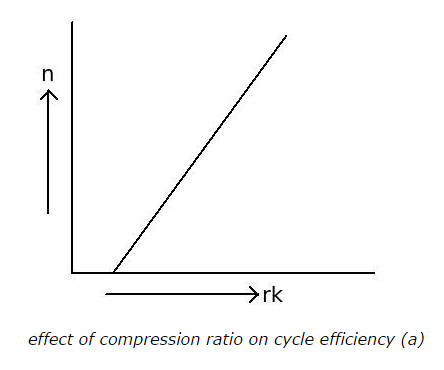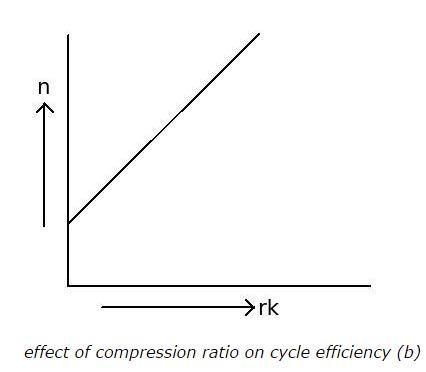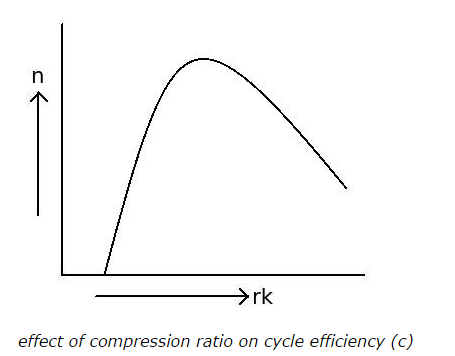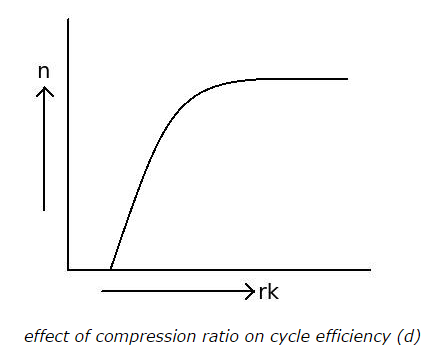# Otto Cycle - MCQs with Answers

## Otto Cycle - MCQs with Answers

Q1. Otto cycle is the air standard cycle of

a. spark ignition (SI) engine
b. compression (CI) ignition engine
c. both SI and CI engines
d. none of the above

ANSWER: a. spark ignition (SI) engine

Q2. How is the heat added in the Otto cycle?

a. reversibly at constant pressure
b. irreversibly at constant pressure
c. reversibly at constant volume
d. irreversibly at constant volume

ANSWER: c. reversibly at constant volume

Q3. What is the formula for compression (rk) ratio of the Otto cycle?

a. rk = Volume of cylinder at the beginning of compression / Volume of cylinder at the end of compression

b. rk = Volume of cylinder at the end of compression / Volume of cylinder at the beginning of compression

c. rk = Volume of cylinder at the end of compression / clearance volume

d. none of the above

ANSWER: a. rk = Volume of cylinder at the beginning of compression / Volume of cylinder at the end of compression

Q4. What is the relation between compression ratio (rk) and the efficiency of the Otto cycle?

a. efficiency decreases with increase in compression ratio
b. efficiency increases with increase in compression ratio
c. efficiency does not affected by change in compression ratio
d. none of the above

ANSWER: b. efficiency increases with increase in compression ratio

Q5. The compression ratio cannot be increased beyond certain limit, because it results to

a. auto-ignition of fuel
b. detonation
c. engine knocking
d. all of the above

ANSWER: d. all of the above

Q6. What should be the correct graph for effect of compression ratio (rk) on cycle efficiency?

a.b.c.d.ANSWER: d.Q7. Use of gasoline blend like gasoline mixed with tetraethyl lead in internal combustion engine

a. increases the octane rating of the fuel
b. allows engine to operate at higher compression ratio
c. avoids auto-ignition of fuel
d. all of the above

ANSWER: d. all of the above

Q8. How is the efficiency of the SI engine affected by change in specific heat ratio (γ) of the working fluid?

a. the efficiency of the engine increases with increase in specific heat ratio (γ) of the working fluid
b. the efficiency of the engine decreases with increase in specific heat ratio (γ) of the working fluid
c. the efficiency of the engine does not affected by change in specific heat ratio (γ) of the working fluid
d. none of the above